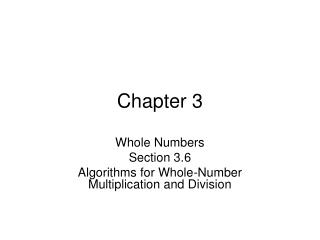Download PresentationChapter 3

# Chapter 3 - PowerPoint PPT PresentationDownload Presentation## Chapter 3

- - - - - - - - - - - - - - - - - - - - - - - - - - - E N D - - - - - - - - - - - - - - - - - - - - - - - - - - -
##### Presentation Transcript

1. Chapter 3 Whole Numbers Section 3.6 Algorithms for Whole-Number Multiplication and Division

2. In this section we look at algorithms for multiplying and dividing whole numbers. Just like the methods for addition and subtraction we will break apart the numbers into the digits that make them up so that we only need to deal with only facts about one digit numbers. Multiplication Algorithms Like addition the table to the right shows the basic multiplication facts. They are first developed by some of the visual models we talked about. They are memorized through repeated use or deliberately by methods such as flash cards. Multiplication algorithms also makes use the idea that multiplying by powers of 10 causes a “shift” in where individual digits are located. The shift in the digits is by determined by what power you multiply by (i.e. the number of zeros) 9  103 = 9  1000 = 9000 and 83  102 = 83  100 = 8300

3. Partial Products Algorithm The standard algorithm for multiplication involves using the basic multiplication facts and the places values the digits are in to determine how many zeros go after the result of each pair of multiplication facts. For Example: find 38  57 50 7 38 57 56 210 400 1500 2166 The numbers come from the following partial products. 30 + 8 50 + 7 8  7 30  7 8  50 30  50 2166 This is represented using an area model as shown. 210 30 1500 8 400 56 This algorithm uses the distributive property repeatedly: 38  57 = (30 + 8)  (50 + 7) = ((30 + 8)  50 + (30 + 8)  7 = 30  50 + 8  50 + 30  7 + 8  7 apply the distributive property of mult over addition apply the distributive property of mult over addition

4. The Lattice Method Another method for multiplying numbers which provides more structure for how you multiply is called the lattice method of multiplication. It uses a diagonally represented table and fills in the entries with the basic multiplication facts. For Example: to do the problem 317  46 = 14582 1. Fill in the corresponding squares with the basic multiplication facts putting the tens digit above the diagonal. 2. Add down each diagonal starting at the bottom right carrying into the next diagonal. 1 1 1 0 2 1 2 4 8 1 0 4 4 8 6 2 5 8 2 14582 3. The final answer you get by taking the digits going down the left side and along the bottom.

5. Division The algorithms for division make use of the repeated subtraction method for division that we discussed earlier. Again, like multiplication we do this in groups of ten. The method used is called the scaffold method. If we think in terms of grouping by tens 246  7 = 35 remainder 1 We can check this by doing the arithmetic problem: 7  35 + 1 = 245 + 1 = 246 The scaffold method is sometimes very difficult and there are many different types of errors students can make when they go to apply it. What is the following error when the scaffold method is applied to the following problems?

6. What would they get for this problem? 397  6 = 661 467  9 = 518 1463  5 = 2923 What is the mistake they are making? They are making two mistakes. The first is an alignment error that occurs at the top of the scaffold. They start the quotient in the incorrect place. The second error is the take the remainder and put it in the ones place.